##### Actions

(diff) ← Older revision | Latest revision (diff) | Newer revision → (diff)

A branch of modern algebra. Its principal task is to represent any ideal of a ring (or of another algebraic system) as the intersection of a finite number of ideals of special type (primary, tertiary, primal, uniserial, etc.). The type of the representation is so chosen that: 1) for any ideal there exists a representation, in other words, some "existence" theorem holds; 2) the representations chosen must be unique apart from certain limitations or, in other words, some "uniqueness" theorem must hold. The fundamental principles of the additive theory of ideals were introduced in the 1920s and the 1930s by E. Noether  and W. Krull .

All special features of the additive theory of ideals are clearly manifested in the case of rings. Let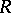be a Noetherian ring, i.e. an associative ring with the maximum condition for ideals. Ifis an ideal of, then there exists a largest ideal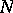offor whichfor some integer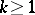. This idealis known as the primary radical of(in) and is denoted by. An idealofis said to be primary if for any two idealsandof, the condition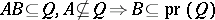is satisfied. The intersection theorem is valid for primary ideals: The intersection of two primary ideals having the same primary radicalis itself a primary ideal with radical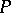. This theorem is used to prove an existence theorem: If the ringis commutative, then for any idealthere exists a representation ofas the intersection of a finite number of primary ideals: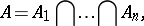(1)

such that none of the ideals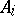contains the intersection of the other ones, and such that the primary radicals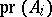are pairwise different. Such representations are known as non-contractible or primarily reduced , . The uniqueness theorem holds for such representations: If (1) holds and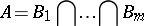(2)

is a second primarily-reduced representation of the idealof the ring, then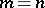and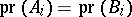for, provided the idealsare suitably renumbered.

The additive theory of ideals of Noetherian commutative rings (the classical additive theory of ideals) has found numerous applications in various branches of mathematics.

If the ringis non-commutative, the above-mentioned existence theorem is no longer valid, but the uniqueness and intersection theorems still hold. This is why, ever since the 1930s, repeated attempts have been made to find a generalization of classical primarity to the non-commutative case such that the existence theorem, too, remains valid. Such a generalization has in fact been found , and is known as tertiarity (cf. Tertiary ideal). It was subsequently shown that, within certain natural limitations, tertiarity is the only "good" generalization of the concept of primarity , , .

During the 1960s the additive theory of ideals developed further within the framework of lattice theory, of systems with fractions and of multiplicative systems , , ; this stimulated the development of the additive theory of ideals for non-associative rings, normal divisors of a group and submodules of a module.

How to Cite This Entry: